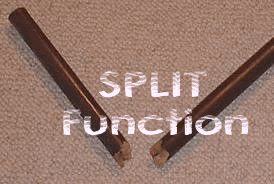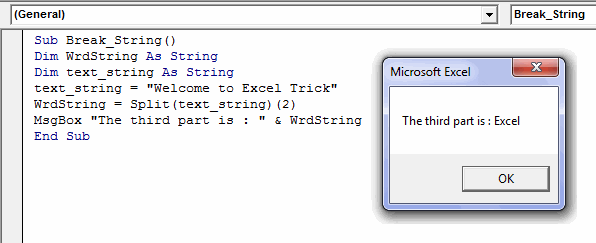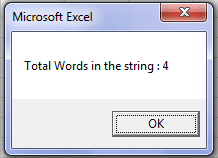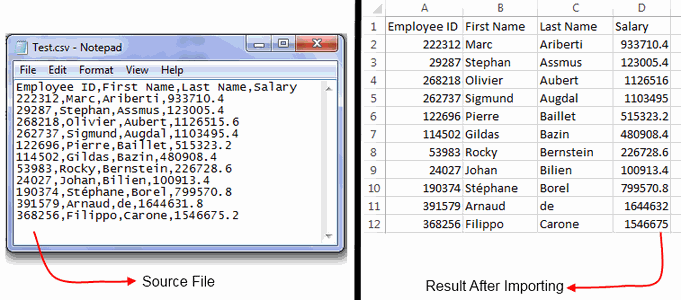# VBA Split Function – How to Use

VBA, just like any other language gives you a set of functions to perform various operations on strings. And VBA Split is one of those string functions. Microsoft introduced Split function with VBA Version 6 (in Office 2000).

As the name suggests, the job of Split statement is to break, split or divide a string based on a particular criteria.

Split is one of the many Substring functions that can be used in VBA. I have written a whole post on these Substring functions, you can read it here.### Definition and Syntax of VBA Split Function:

Split can be defined as a function that can split a text string into an array, by making use of a delimiter character. It returns a zero-based, one-dimensional array holding the parts of the original text string.

The Syntax of  VBA Split Statement is as follows:

`Split (text_string, delimiter, limit, compare)`

Here, ‘`text_string`’ refers to the string that you need to break.

`delimiter`’ refers to the character which divides the string into parts. This is an optional argument, space character “ ” is treated as the default delimiter.

`limit`’ is an optional parameter. It specifies the maximum number of parts into which the input string should be divided. The default value is -1, which means that the string should be divided at each occurrence of ‘`delimiter`’.

`compare`’ is also an optional argument that specifies the comparison method to be used while evaluating the strings. ‘`compare`’ argument can have anyone of the below values:

ConstantValueDescription
CompareMethod.Binary0Performs a binary comparison i.e. each character should only match itself.
CompareMethod.Text1Performs a textual comparison.

### Some Important points about Split Function:

• Split Function returns a String Array and not a String.
• If you omit the ‘compare’ argument then, Split Statement uses the Binary comparison method as default.
• If the ‘text_string’ that you pass to Split is a zero length string then, it returns a single-element array containing a zero-length string.
• If the ‘delimiter’ is not found anywhere in the ‘text_string’, then Split returns the single-element array containing ‘text_string’ as it is.

### How to Use VBA Split Function:

Ok, now let’s see how you can use the Split function:

Objective: Let’s consider we have a string : “How are you” and now our objective is to break this string to separate out the words.

So, we will try to apply a Split Statement to it as:

`Split (text_string, delimiter, limit, compare)`

`text_string`’: In this case our ‘text_string’ would be “How are you”.

`delimiter`’: In this case our delimiter would be space character (“ ”). But as I have foretold that, space character is the default delimiter, so will leave this argument blank.

`limit`’: We will also leave the ‘limit’ argument blank because we need to separate out all the words from the given ‘text_string’.

`compare`’: This would be blank, as blank specifies binary comparison method.

So, the final Split Function would be:

`Split("Hello how are you")`

Note: Now we need to receive the return from this split function, for this purpose we can use a string array.

So, the final code would be:

`Sub TestCode()Dim WrdArray() As StringWrdArray() = Split("How are you")End Sub`

### 5 Examples using Split Function:

Now let’s move on to some examples of Split Statements:

Example 1: Separate a list of pipe separated values i.e. A|B|C|D.

Below is the code to do this:

`Sub Break_String()Dim WrdArray() As StringDim text_string As Stringtext_string = "A|B|C|D"WrdArray() = Split(text_string, "|")End Sub`

Example 2: Break the string (say: “Welcome to Excel Trick”) into separate words by using Split Function. And display the contents of the array returned by the Split using a message box.

Below is the code that can accomplish this task:

`Sub Break_String()Dim WrdArray() As StringDim text_string As Stringtext_string = "Welcome to Excel Trick"WrdArray() = Split(text_string)For i = LBound(WrdArray) To UBound(WrdArray) strg = strg & vbNewLine & "Part No. " & i & " - " & WrdArray(i)Next iMsgBox strgEnd Sub`Explanation:

In this code, we have used a Split function to break the string into parts. Later, in the code we have used a For loop to iterate the array returned by the Split Function in order to show the array contents using a message box.

Example 3: Use the above example, but here after splitting the string we just need to display its third part. To make it clearer, let’s say if I divide the string (“Welcome to Excel Trick”), then in this case I just need the output as “Excel” i.e. the third word in the string.

So, the code would be:

`Sub Break_String()Dim WrdString As StringDim text_string As Stringtext_string = "Welcome to Excel Trick"WrdString = Split(text_string)(2)MsgBox "The third part is : " & WrdStringEnd Sub`Explanation:

In this code, you would have noticed that instead of using a String Array for accepting the return from the Split Function I have taken a string variable. This is because by writing the Split Statement as: `WrdString = Split(text_string)(2)`, we are telling it to return only the third element of the array (which is a string) and not the complete array.

Example 4: Now use the same code of Example 2 just change it to demonstrate the use of limit argument.

`Sub Break_String()Dim WrdArray() As StringDim text_string As Stringtext_string = "Welcome to Excel Trick"WrdArray() = Split(text_string, , 3)For i = LBound(WrdArray) To UBound(WrdArray) strg = strg & vbNewLine & "Part No. " & i & " - " & WrdArray(i)Next iMsgBox strgEnd Sub`Explanation:

Above code is very similar to the one that we have seen in Example 2. The only difference here is that we have supplied the limit argument to Split function.

Notice: Because of the limit argument, Split function divides the text string into 3 parts instead of 4.

Example 5: Write a code using Split function that can count the number of words in a string.

Below is the code that can do this:

`Sub Count_Words()Dim WrdArray() As StringDim text_string As Stringtext_string = "Welcome to Excel Trick"WrdArray() = Split(text_string)MsgBox ("Total Words in the string : " & UBound(WrdArray()) + 1)End Sub`Explanation:

In this example, we have used the UBound function to get the upper bound of `WrdArray()`. The statement `UBound(WrdArray())` gives the length of `WrdArray()`. But as we all know, that an array starts from index 0, so we need to add 1 to its upper bound to get the actual word count.

Example 6: Write a code that can import a CSV to a spreadsheet.

This code requires you to understand the basics of FileSystemObject. So, before moving ahead I would recommend you to read this post.Below is the code to do this:

`'Creating a FileSystemObjectPublic FSO As New FileSystemObjectSub Import_CSV()Dim WrdArray() As StringDim txtstrm As TextStreamDim line As StringDim clm As LongDim Rw As LongSet txtstrm = FSO.OpenTextFile("C:\Users\Ankit\ExcelTrick\Test.csv")Rw = 1Do Until txtstrm.AtEndOfStream line = txtstrm.ReadLine clm = 1 WrdArray() = Split(line, ",") 'Change with ; if required For Each wrd In WrdArray() ActiveSheet.Cells(Rw, clm) = wrd clm = clm + 1 Next wrd Rw = Rw + 1Looptxtstrm.CloseMsgBox "Data Imported. " & Rw & " Records Found."End Sub`Explanation:

As we know that CSV files are comma separated files. In this example, we are reading each line from a pre-existing CSV file and then by using split function we are separating individual values from each line and writing them to the Active Sheet.

So, this was all about VBA Split Function from my side. Feel free to drop in your comments related to the topic.About Ankit Kaul

Ankit is the founder of Excel Trick. He is tech Geek who loves to sit in front of his square headed girlfriend (his PC) all day long. :D. Ankit has a strong passion for learning Microsoft Excel. His only aim is to turn you guys into 'Excel Geeks'.